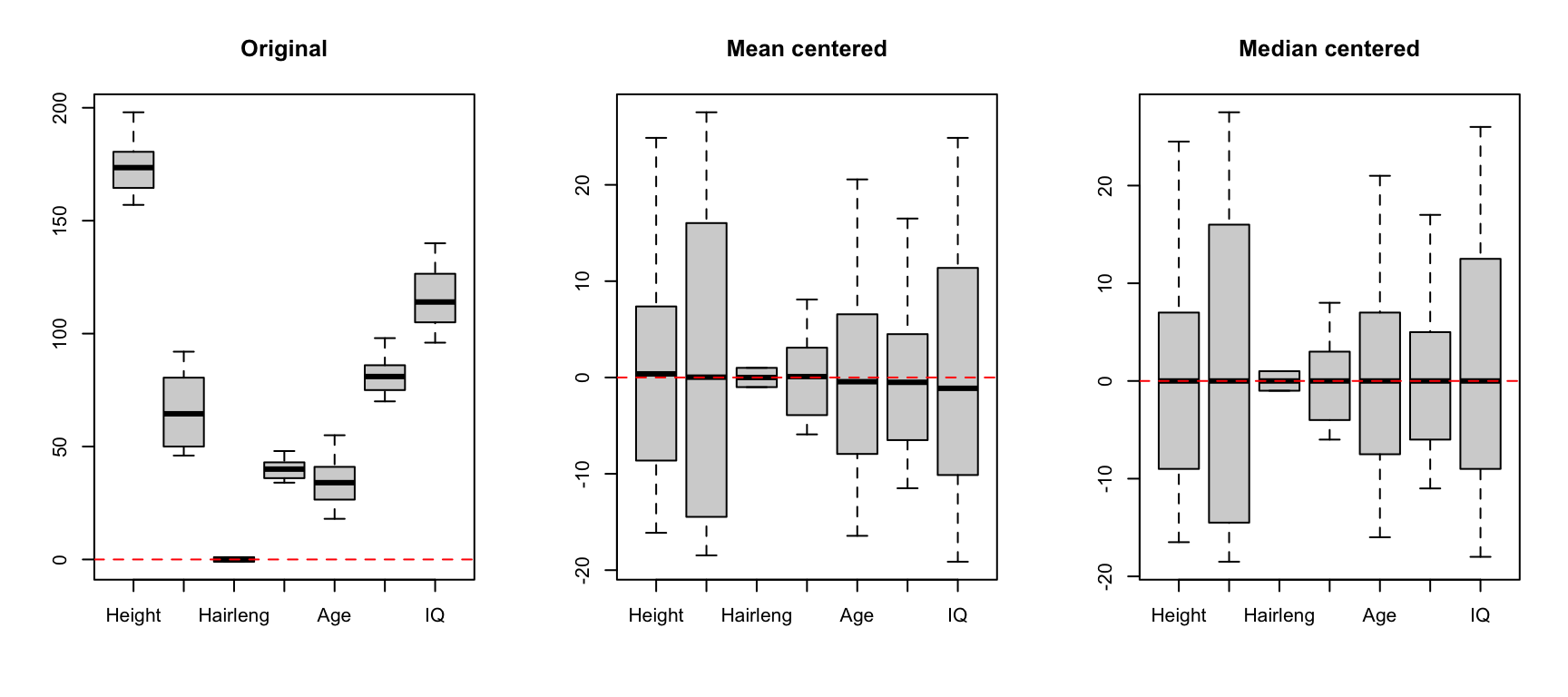## Smoothing and derivatives

Savitzky-Golay filter is used to smooth signals and calculate derivatives. The filter has three arguments: a width of the filter (width), a polynomial order (porder) and the derivative order (dorder). If the derivative order is zero (default value) only smoothing will be performed.

The next chunk of code takes the spectra from Simdata, adds additional random noise using random numbers generator for normal distribution and then applies the SG filter with different settings. The results are shown as plots under the chunk.

# load UV/Vis spectra from Simdata
data(simdata)
ospectra = simdata$spectra.c attr(ospectra, "xaxis.values") = simdata$wavelength
attr(ospectra, "xaxis.name") = "Wavelength, nm"

# add random noise to the spectra
nspectra = ospectra + 0.025 * matrix(rnorm(length(ospectra)), dim(ospectra))

# apply SG filter for smoothing
pspectra = prep.savgol(nspectra, width = 15, porder = 1)

# apply SG filter for smoothing and take a first derivative
dpspectra = prep.savgol(nspectra, width = 15, porder = 1, dorder = 1)

# show results
par(mfrow = c(2, 2))
mdaplot(ospectra, type = "l", main = "Original")
mdaplot(nspectra, type = "l", main = "Noise added")
mdaplot(pspectra, type = "l", main = "Smoothing")
mdaplot(dpspectra, type = "l",main = "First derivative")Starting from v.0.12.0 the algorithm has been modified in order to treat the end points better (for example, when a derivative is taken the end points may look a bit weird and have to be truncated). The implemented algorithm is based on the method described in this article.